# The network output matches the target curve but with a different scale

I have a simple feed-forward net with one input and one output, it runs but when I graph the target versus the network output, the two curves matches but they are on different scale

here is the graph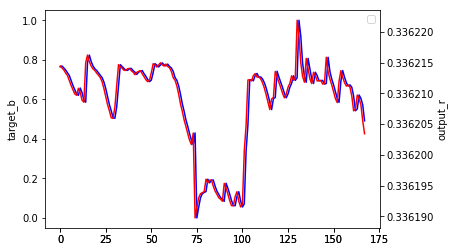thanks

What is your model supposed to learn?
Is it just a simple linear mapping of the form `f(x) = w*x+b` without any hidden layers or are you using multiple layers?
Could you post the value ranges of the input and output?
Maybe your model just didn’t finish to learn the scaling.
Normalizing the output might help, but let’s first see, what you are dealing with.

Im passing yesterday’s groundwater level as input and getting today’s groundwater level as an output. there is one hidden layer with two nodes, im using ReLU for the hidden layer and Sigmoid for the output layer. I normalized both the input and the output.
what I am trying to do here is to test if the model actually works as this is the my first model ever, and it must converge because I am using the same data for input and output except that the output is the input shifted one day ahead and with groundwater level; we are talking about centimeters difference between today and yesterday. so the data is time series water level, the same series is shifted one day and used as an output.

Thanks for the info!
Could you post the loss curve?
I think your model might got into a saddle point and did not converged yet.

thank you so much for replying, here is the loss curvebut I have to say that I managed to improve the output values and their scale by tweaking the weights, here is the better output (testing set) values versus target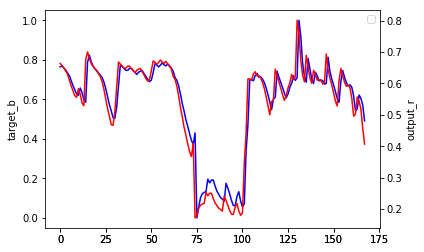but still the network does not hit the highest and the lowest values, to clarify better, here is the plot of the training set output versus target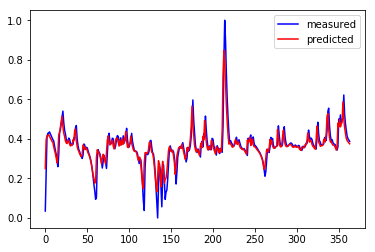It looks like the training loss is going down quite steeply. Did the validation loss increase or why did you stop the training?

because the loss does not decrease anymore on the training set, even if i change the learning rate. on the testing set, the loss is decreasing. I didn’t use a validation set, yet I am using the testing loss with every epoch to show if i am over fitting or not, and to see in general what is happening.
(edit), here is the result if i run it for more epochs,ruined again and cannot get a bigger output scale

Based on the images I’m not sure your model is currently learning anything.
The output still looks like the shifted version of the target, i.e. basically the scaled input.
Could you plot the output and input into a single plot to see if this effect is really there?

here it is for the testing set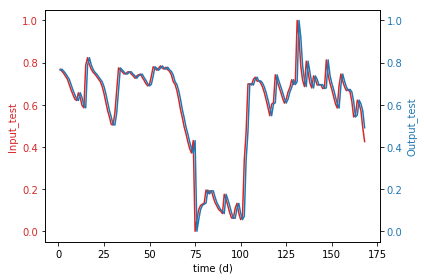its learning cause at the beginning of the training, i get different shapes, with some tweaks with the hyper parameters, I manage to get what i sent before.

maybe this could give you a better idea, i tried different parameters, and i got the following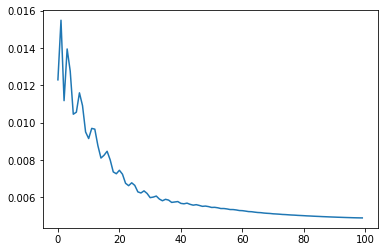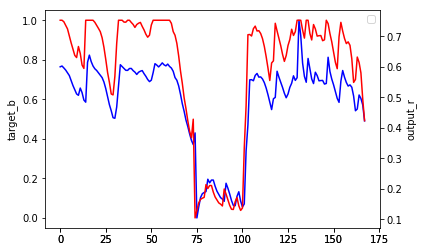and by the end of today, i managed to get this:so, do you think its learning based on this! thank you

Looks quite good by now.
Do you know why the loss shows these waves? Is it related to a new epoch or are you playing around with the learning rate?

I set the network to generate weights randomly following normal distribution of mean = 0 and Stdev =1, trained it at first with Adam. then i saved the weights and went on with training using SGD, and thats when i started to see this wavy loss, so I assume its form the momentum and the learning rate.
(edit) worth mentioning that, I got this wavy loss without changing the learning rate and the momentum, i just their values and run the model for a 1000 epoch and i got the curve above.

Try a linear activation at the output instead of the Sigmoid.

I tried that with ReLU but i could not get a final good result. thanks for the suggestion though

The two curves here are almost fully correlated with each other but they have different scales. So, my guess is the loss function you have used is somehow related to correlation. But correlation does not take care of the scale, so it explains why the scales of input and output are different.

(1) Are you using dataloader, you should turn on shuffle (creating a batch of random training examples at each iteration)
(2) You said input was yesterday’s groundwater level and output was today’s groundwater level? Assuming groundwater level in a short period of time is similar, and there is no signal to predict increasing or decreasing groundwater level, then the best prediction would be the same groundwater level value from yesterday.
(3) Your network should be able to learn to make such prediction. If not you are having some bugs in your code. Another possibility is that your testing data distribution is different from training, try visualize them as histograms